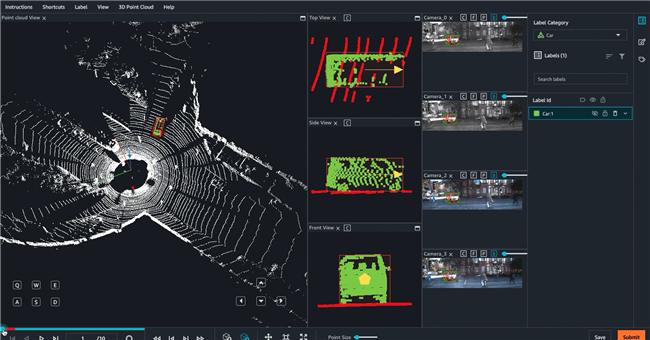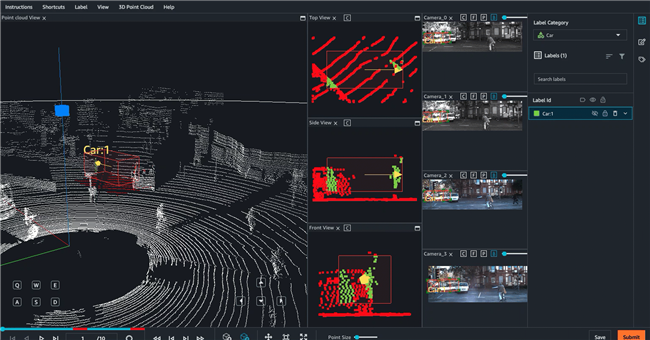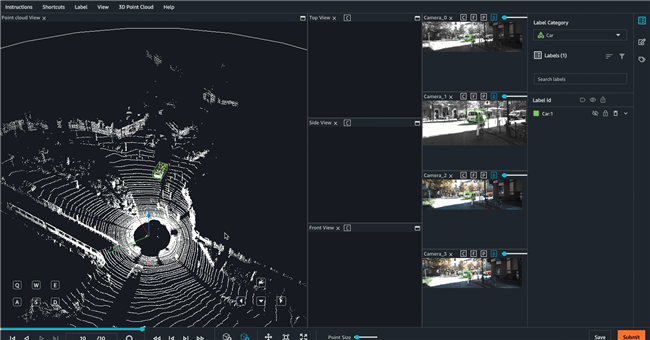# 在 Amazon SageMaker Ground Truth 中标记数据，以实现 3D 对象跟踪与传感器融合

Original URL: https://aws.amazon.com/cn/blogs/machine-learning/labeling-data-for-3d-object-tracking-and-sensor-fusion-in-amazon-sagemaker-ground-truth/

Amazon SageMaker Ground Truth 现在支持对3D点云数据进行标记。关于其他功能的更多详细信息，请参阅AWS News博客。在本文中，我们将专门介绍如何对3D点云数据执行数据转换，从而使用SageMaker Ground Truth来标记3D对象跟踪。

SageMaker Ground Truth能够轻松在一系列3D点云帧上标记对象以构建机器学习训练数据集，且支持将多达8台摄像机输入的LiDAR传感数据加以融合。SageMaker Ground Truth做图像融合要求视频帧与3D点云帧进行预先同步。在启用传感器融合功能之后，标记人员可以配合同步视频帧查看3D点云帧。除了为标记工作提供更多视觉环境信息之外，传感器融合功能还会将3D点云中绘制的任何标签投射至视频帧，保证在某一帧内完成的标记调整将准确出现在另一帧中。

• 明确SageMaker Ground Truth 3D点云标记作业的输入数据格式与要求。
• 将KITTI数据集从局部坐标系转换为世界坐标系。
• 将视频数据与同步LiDAR数据相关联，以进行传感器融合。
• 准备一个输入SageMaker Ground Truth 的 manifest文件。
• 为3D点云对象检测创建一个标记作业，并跨越一系列帧进行跟踪。
• 在工作人员标记UI界面当中使用辅助标记工具。

## 3D点云标记作业输入数据

### 传感器融合

LiDAR传感器与每台摄像机都拥有自己的外部矩阵，SageMaker Ground Truth利用它们来实现传感器融合功能。要将标签从3D点云投射至摄像机平面图像，SageMaker Ground Truth需要将3D点由LiDAR坐标系转换为摄像机坐标系。这一转换通常是使用LiDAR外部矩阵将3D点从LiDAR自有坐标转换为世界坐标系来完成的。接下来，我们使用摄像机外部矩阵的逆（从世界到摄像机）将上一步中获得的世界坐标系 3D点转换为摄像机平面图像。如果您的3D点云数据已经转换为世界坐标系形式，则无需进行第一次转换，而且3D与2D之间的转换将仅需要使用摄像机外部矩阵。

``````rotation = [[ 9.96714314e-01, -8.09890350e-02,  1.16333982e-03],
[ 8.09967396e-02,  9.96661051e-01, -1.03090934e-02],
[-3.24531964e-04,  1.03694477e-02,  9.99946183e-01]]

origin= [1.71104606e+00
5.80000039e-01
9.43144935e-01]

from scipy.spatial.transform import Rotation as R
# position is the origin
position = origin
r = R.from_matrix(np.asarray(rotation))
# heading in WCS using scipy

``````import numpy as np

transformation
= [[ 9.96714314e-01, -8.09890350e-02,  1.16333982e-03, 1.71104606e+00],
[ 8.09967396e-02,  9.96661051e-01, -1.03090934e-02, 5.80000039e-01],
[-3.24531964e-04,  1.03694477e-02,  9.99946183e-01, 9.43144935e-01],
[              0,               0,               0,              1]]

transformation  = np.array(transformation )
rotation = transformation[0:3][0:3]
translation= transformation[0:3]

from scipy.spatial.transform import Rotation as R
# position is the origin translation
position = translation
r = R.from_matrix(np.asarray(rotation))
# heading in WCS using scipy

``````{
"position": {
"y": -152.77584902657554,
"x": 311.21505956090624,
"z": -10.854137529636024
},
"qy": -0.7046155108831117,
"qx": 0.034278837280808494,
"qz": 0.7070617895701465,
"qw": -0.04904659893885366
}
}``````

#### 摄像机校准、内部与失真

``````# intrinsic paramaters
fx (float) - focal length in x direction.
fy (float) - focal length in y direction.
cx (float) - x coordinate of principal point.
cy (float) - y coordinate of principal point.
k1 (float) - Radial distortion coefficient.
k2 (float) - Radial distortion coefficient.
k3 (float) - Radial distortion coefficient.
k4 (float) - Radial distortion coefficient.
# Tangential distortion parameters
p1 (float) - Tangential distortion coefficient.
p2 (float) - Tangential distortion coefficient.``````

``````|x|   |f_x, 0, c_x|   |X|
|y| = |0, f_y, c_y| * |Y|
|z|   | 0,  0,  1 |   |Z|``````

### 输入manifest文件

Ground Truth采用输入manifest文件，其中的每一行都描述了需要由注释人员（或者对某些内置任务类型进行自动标记）完成的任务单元。输入manifest文件的格式由您的实际任务类型决定：

• 3D点云对象检测或者语义分段标记作业——输入manifest文件中的每一行，都包含与单一3D点云帧及传感器融合数据相关的信息。此manifest文件被称为3D点云帧输入manifest。
• 3D点云对象检测与标记作业跟踪——输入manifest文件中的每一行都包含指向某一序列文件的链接，该文件负责定义一系列与3D点云帧及传感器融合瞻前顾后 数据。其中各序列被称为3D点云序列，而此manifest被称为点云序列输入manifest。

## 将KITTI数据集转换为世界坐标系

``````import pykitti
import numpy as np

basedir = '/Users/nameofuser/kitti-data'
date = '2011_09_26'
drive = '0079'

# The 'frames' argument is optional - default: None, which loads the whole dataset.
# Calibration, timestamps, and IMU data are read automatically.
# Camera and velodyne data are available via properties that create generators
# when accessed, or through getter methods that provide random access.
data = pykitti.raw(basedir, date, drive, frames=range(0, 50, 5))

# i is frame number
i = 0

# customer computes lidar extrinsics
lidar_extrinsic_matrix = data.oxts[i].T_w_imu

# velodyne raw point cloud in lidar scanners own coordinate system
points = data.get_velo(i)

# transform points from lidar to global frame using lidar_extrinsic_matrix
def generate_transformed_pcd_from_point_cloud(points, lidar_extrinsic_matrix):
tps = []
for point in points:
transformed_points = np.matmul(lidar_extrinsic_matrix, np.array([point, point, point, 1], dtype=np.float32).reshape(4,1)).tolist()
if len(point) > 3 and point is not None:
tps.append([transformed_points, transformed_points, transformed_points, point])

return tps

# customer transforms points from lidar to global frame using lidar_extrinsic_matrix
transformed_pcl = generate_transformed_pcd_from_point_cloud(points, lidar_extrinsic_matrix)``````

## 将视频数据与LiDAR数据相关联，以实现传感器融合

KITTI提供LiDAR外部与相机外部矩阵。您可以使用这些矩阵提取姿态数据，而后根据3D点云序列输入manifest的需求将这部分数据转换为JSON格式。

``````from scipy.spatial.transform import Rotation as R

# utility to convert extrinsic matrix to pose heading quaternion and position
def convert_extrinsic_matrix_to_trans_quaternion_mat(lidar_extrinsic_transform):
position = lidar_extrinsic_transform[0:3, 3]
rot = np.linalg.inv(lidar_extrinsic_transform[0:3, 0:3])
quaternion= R.from_matrix(np.asarray(rot)).as_quat()
trans_quaternions = {
"translation": {
"x": position,
"y": position,
"z": position
},
"rotation": {
"qx": quaternion,
"qy": quaternion,
"qz": quaternion,
"qw": quaternion
}
}
return trans_quaternions``````

``````def convert_camera_inv_extrinsic_matrix_to_trans_quaternion_mat(camera_extrinsic_transform):
position = camera_extrinsic_transform[0:3, 3]
rot = np.linalg.inv(camera_extrinsic_transform[0:3, 0:3])
quaternion= R.from_matrix(np.asarray(rot)).as_quat()
trans_quaternions = {
"translation": {
"x": position,
"y": position,
"z": position
},
"rotation": {
"qx": quaternion,
"qy": quaternion,
"qz": quaternion,
"qw": -quaternion
}
}
return trans_quaternions``````

### 相机校准：内部与失真

KITTI数据集为每台摄像机提供一项校准参数。例如，`data.calib.K_cam0`当中就包含以下相机内部矩阵：

``````            fx 0  cx
0  fy cy
0  0   1``````

## 创建输入manifest文件

``````def convert_to_gt():
# The 'frames' argument is optional - default: None, which loads the whole dataset.
# Calibration, timestamps, and IMU data are read automatically.
# Camera and velodyne data are available via properties that create generators
# when accessed, or through getter methods that provide random access.
data = pykitti.raw(basedir, date, drive, frames=range(0, 50, 5))
image_paths  = [data.cam0_files,  data.cam1_files, data.cam2_files, data.cam3_files]
camera_extrinsic_calibrations = [data.calib.T_cam0_velo, data.calib.T_cam1_velo, data.calib.T_cam2_velo, data.calib.T_cam3_velo]
camera_intrinsics = [data.calib.K_cam0, data.calib.K_cam1, data.calib.K_cam2, data.calib.K_cam3]
seq_json = {}
seq_json["seq-no"] = 1
seq_json["prefix"] = 's3://pdx-groundtruth-lidar-test-bucket/pdx-groundtruth-sequences/kittiseq2/frames/'
seq_json["number-of-frames"] = len(data)
seq_json["frames"] = []
default_position = {"x": 0, "y": 0, "z": 0}
default_heading = {"qx": 0, "qy": 0, "qz": 0, "qw": 1}
for i in range(len(data)):
# customer computes lidar extrinsics
lidar_extrinsic_matrix = data.oxts[i].T_w_imu
# velodyne raw point cloud in lidar scanners own coordinate system
points = data.get_velo(i)
# customer transforms points from lidar to global frame using lidar_extrinsic_matrix
transformed_pcl = generate_transformed_pcd_from_point_cloud(points, lidar_extrinsic_matrix)
# Customer computes rotation quaternion and translation from LiDAR Extrinsic
trans_quaternions = convert_extrinsic_matrix_to_trans_quaternion_mat(lidar_extrinsic_matrix)
# Customer uses trans_quaternions to populates GT ego veicle pose
ego_vehicle_pose = {}
ego_vehicle_pose['position'] = trans_quaternions['translation']
# open file to write the transformed pcl
with open(output_base+"/"+str(i)+'.txt', "w") as out:
writer = csv.writer(out, delimiter=' ', quotechar='"', quoting=csv.QUOTE_MINIMAL)
for point in transformed_pcl:
writer.writerow((point, point, point, point))
frame = {}
frame["frame-no"] = i
frame["frame"] = str(i)+'.txt'
frame["unix-timestamp"] = data.timestamps[i].replace(tzinfo=timezone.utc).timestamp()
frame["ego-vehicle-pose"] = ego_vehicle_pose
images = []
image_dir_path = os.path.join(output_base, 'images')
if not os.path.exists(image_dir_path):
os.makedirs(image_dir_path)
for j in range(len(image_paths)):
# copy image
image_path = image_paths[j][i]
image_suffix_path = 'images/frame_'+str(i)+'_camera_'+str(j)+'.jpg'
copyfile(image_path, os.path.join(output_base, image_suffix_path))
# If customer has the camera extrinsics then they use them to compute the camera transform
camera_transform= np.linalg.inv(np.matmul(camera_extrinsic_calibrations[j], np.linalg.inv(data.oxts[i].T_w_imu)))
# Customer computes rotation quaternion and translation from camera inverse Extrinsic
cam_trans_quaternions = convert_camera_inv_extrinsic_matrix_to_trans_quaternion_mat(camera_transform)
image_json = {}
image_json["image-path"] = image_suffix_path
image_json["unix-timestamp"] = frame["unix-timestamp"]
image_json['position'] = cam_trans_quaternions['translation']
image_json['fx'] = camera_intrinsics[j]
image_json['fy'] = camera_intrinsics[j]
image_json['cx'] = camera_intrinsics[j]
image_json['cy'] = camera_intrinsics[j]
image_json['k1'] = 0
image_json['k2'] = 0
image_json['k3'] = 0
image_json['k4'] = 0
image_json['p1'] = 0
image_json['p2'] = 0
image_json['skew'] = 0
images.append(image_json)
frame["images"]=images
seq_json["frames"].append(frame)
with open(output_base+'/mykitti-seq2.json', 'w') as outfile:
json.dump(seq_json, outfile)``````

## 创建标记作业

• 标签自动填充——当工作人员向帧中添加框体时，此功能会自动将框体请回至序列中的所有帧。当工作人员手动调整其他帧中同一对象的注释时，自动填充标签也将进行自动调整。
• 标签插值——工作人员在两个帧中标记单一对象后，Ground Truth会使用这些注释在两帧之间的移动中对该对象进行插值。
• 捕捉——工作人员可以在对象周边添加一个框体，并使用键盘快捷键或菜单选项让Ground Truth自动拟合工具紧紧贴合对象的边界。
• 适应地面——在工作人员向3D场景中添加框体后，工作人员可以自动使该框体与地面相适应。例如，工作人员可以使用此功能将框体贴合至场景中的道路或人行道。
• 多视图标记——工作人员将3D框体添加至3D场景之后，侧面板上会显示正面与侧面两个透视图，以帮助工作人员在对象周边紧密调整该框体。工作人员可以在3D点云或侧面板上调整标签，相应调整也会实时显示在其他视图当中。
• 传感器融合——如果您提供传感器融合数据，则工作人员可以在3D场景与2D图像当中调整注释，且该注释会被实时投射至其他视图当中。Ground Truth每15分钟自动保存一次注释。在作业完成之后，选择Submit。当任务完成后，输出数据将被保存于您在`HumanTaskConfig`中指定的Amazon Simple Storage Service (Amazon S3)存储桶当中。

## 总结

 KITTI数据集拥有自己的使用许可。请保证您对数据集的使用方式完全符合其中提出的条款与要求。

## 本篇作者Vikram Madan 是 Amazon SageMaker Ground Truth 产品经理。他专注于交付可以更轻松构建机器学习解决方案的产品。闲暇时间，他喜欢长跑和观看纪录片。### Zahid Rahman

AWS AI高级软件开发工程师。他主要负责开发复杂的机器学习服务与大规模分布式系统。他对计算机视觉、人工智能以及大数据技术抱有浓厚兴趣。在业余时间，他喜欢阅读历史书籍。